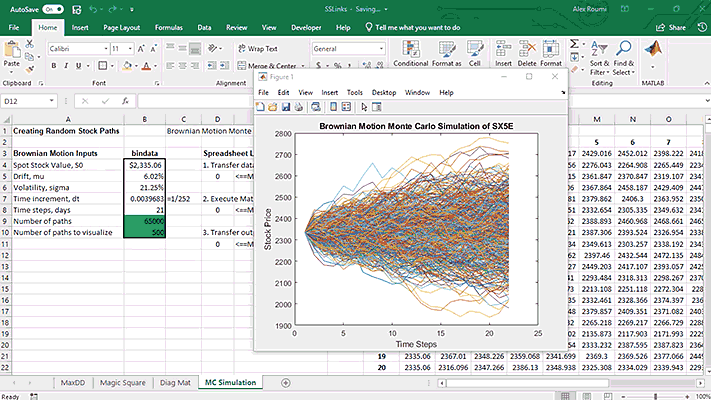Interactively access MATLAB capabilities from the MATLAB group menu in Excel.### MATLAB Functions in Microsoft Excel

Execute built-in and custom functions in MATLAB directly from Excel or via Visual Basic for Applications (VBA) Macros.### Exchanging Data Between Excel and MATLAB

Transfer data between Excel and MATLAB in both directions using the MATLAB group menu in Excel, VBA macros, or the Spreadsheet Link toolbar.

#### Product Resources:

“When you deal with numbers all day and work with sophisticated analytical models, having an integrated environment is invaluable. With MATLAB we visualize data, conduct back-testing, and plot graphs to see the results of changes we make, all in one environment, and that saves time.”

Simone Visonà, A2A